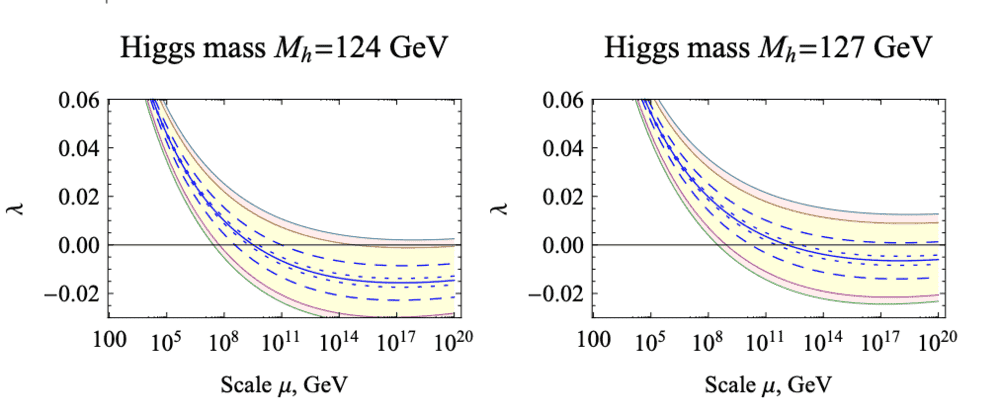# Claimed derivation of Weinberg angle, Higgs/W mass ratio

Gold Member
https://arxiv.org/abs/2010.15621
Superselection of the weak hypercharge and the algebra of the Standard Model

Ivan Todorov
[Submitted on 29 Oct 2020]
We postulate that the exactly conserved weak hypercharge Y gives rise to a superselection rule for both observables and gauge transformations. This yields a change of the definition of the particle subspace adopted in recent work with Michel Dubois-Violette; here we exclude the zero eigensubspace of Y consisting of the sterile (anti)neutrinos which are allowed to mix. One thus modifies the Lie superalgebra generated by the Higgs field. Equating the field normalizations in the lepton and the quark subalgebras we obtain a relation between the masses of the W boson and the Higgs that fits the experimental values within one percent accuracy.

I haven't had time to study this paper yet. But a few curiosities:

It talks about Clifford algebras. But in fact it builds on work due to Michel Dubois-Violette, who comes from noncommutative geometry but who somehow uses the 27-dimensional exceptional Jordan algebra as the noncommutative space in his physics model.

It talks about superalgebras. But it looks like the concept of "super" here can't be the usual one from particle physics. Indeed, I suspect that the author is really talking about a "Z2-graded algebra" rather than a true "superalgebra" (both algebras have "even" and "odd" objects, but only in the true superalgebra are the odd objects fermionic).

The main thing I wish to understand, is how the author obtains the Weinberg angle and W/Higgs mass ratio. But one probably needs to grasp the overall theoretical framework too, to really understand.

•ohwilleke and atyy

Mentor
Cant' comment on the overall calculations but I can check 4.17. There are two claims:

1) ##\frac 5 8 = \cos^2(\theta_W)## which implies ##\frac 3 8 = \sin^2(\theta_W)##
CODATA 2018 tells us ##\sin^2(\theta_W)=0.22290(30)## which isn't anywhere close to 3/8 = 0.375.

2) ##m_H=2\cos(\theta_W)m_W##
PDG has the W mass at 80.379±0.012 GeV and 91.1876±0.0021 GeV. Using the experimental Weinberg angle we get ~141 GeV which is obviously absurd. Using ##\sqrt{\frac{5}{8}}## we get 127.090 +- 0.019 GeV. That's 14 standard deviations away from the experimental result (using PDG's 125.10 +- 0.14 GeV).

Good, experiments ruled out their model.

•ohwilleke, atyy and mitchell porter
Gold Member
Thanks for pointing out this paper! I wrote about how Dubois-Violette and Todorov got the Standard Model gauge group from the exceptional Jordan algebra, and I'm just about to explain in that in a lot more detail, but I hadn't seen this brand-new paper.

A lot of grand unified theories predict ##\sin^2 (\theta_W) = 3/8## at very high energies; then one needs to do renormalization group calculations to compute ##\sin^2 (\theta_W)## at collider energies. (Remember, coupling constants depend on the energy scale.) So, I don't think a high-energy prediction of ##\sin^2 (\theta_W) = 3/8## is death to a theory.

The abstract of this paper shows how flexible string theory has become:
We investigate whether the hypercharge assignments in the Standard Model can be interpreted as a hint at Grand Unification in the context of heterotic string theory. To this end, we introduce a general method to calculate U(1)_Y for any heterotic orbifold and compare our findings to the cases where hypercharge arises from a GUT. Surprisingly, in the overwhelming majority of 3-2 Standard Models, a non-anomalous hypercharge direction can be defined, for which the spectrum is vector-like. For these models, we calculate sin^2 theta to see how well it agrees with the standard GUT value. We find that 12% have sin^2 theta = 3/8, while all others have values which are less. Finally, 89% of the models with sin^2 theta = 3/8 have U(1)_Y in SU(5).

When you start talking about percentages of models, it means you have a lot of models!

Last edited:
•ohwilleke, Greg Bernhardt, atyy and 1 other person
Mentor
So, I don't think a high-energy prediction of ##\sin^2 (\theta_W) = 3/8## is death to a theory.
That still leaves the Higgs mass discrepancy. Unless we are supposed to evaluate the formula at an unspecified energy scale. Sure, if the Weinberg angle changes continuously there will be some energy scale (range) where you get the right mass, but if that scale doesn't come from a theoretical motivation it is just numerology.

•ohwilleke and Tendex
•